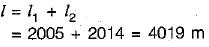Courses

# Linear Measurement - 2

## 10 Questions MCQ Test Mock Test Series for Civil Engineering (CE) GATE 2020 | Linear Measurement - 2

Description
This mock test of Linear Measurement - 2 for Civil Engineering (CE) helps you for every Civil Engineering (CE) entrance exam. This contains 10 Multiple Choice Questions for Civil Engineering (CE) Linear Measurement - 2 (mcq) to study with solutions a complete question bank. The solved questions answers in this Linear Measurement - 2 quiz give you a good mix of easy questions and tough questions. Civil Engineering (CE) students definitely take this Linear Measurement - 2 exercise for a better result in the exam. You can find other Linear Measurement - 2 extra questions, long questions & short questions for Civil Engineering (CE) on EduRev as well by searching above.
QUESTION: 1

### The length of a line measured with a 20 metre chain was found to be 250 metres. What is the true length of line if the chain was 10 cm too long

Solution:

The true length of fine is given by,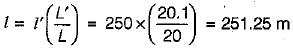QUESTION: 2

### During the measurement of a line by chain or tape in slopes, if the length of line is ‘l’ and height difference between the ends of the line is ‘h’ then the correction to the measured length is more than h2/2l by

Solution:

Cslope = Actual length - Measured length.
= sqrt(L^2 - H^2) - L.

= L x [1- (H^2 / L^2)]^0.5 - L = { L [ 1- 0.5*(H^2 / L^2) + [0.5*(0.5-1)*(H^2 / L^2)^2 / 2] } - L.
= L - L* 0.5*(H^2 / L^2) + L* [0.5*(0.5-1)*(H^2 / L^2)^2 / 2 - L.
= -(H^2)/2L - (H^4)/8*L^3.
Hence, correction to the measured length is more than by (H^4)/8*L^3.

QUESTION: 3

### The required slope correction for a length of 60 m along a gradient of 1 in 20 is

Solution: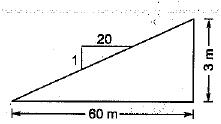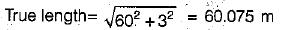Correction = 0.075 m = 7.5 cm

QUESTION: 4

A 30 m steel tape was standardized at 20°C and measurements of distances were taken at 15°C. if the coefficient of linear expansion α of the material of the tape were 0.000112 per °C, then error due to temperature per tape length would be

Solution:

Explanation : The formula for correction C=α(tm -t)L.

So,α= 0.0000112.

Also l= 30m.

Also, temp measured = 15 degrees.

And temp star = 20 degree.

Solving we get -0.001680 but in the question it

Is for per metre tape length so divide by 30.

We get a correction - 0.0000560 but he has asked for error so + 0.0000560 is the correct answer.

QUESTION: 5

A 100 m tape is suspended between the ends under a pull of 200 N. The weight of the tape is 30 N. The correction for sag and correct distance between the tape ends is

Solution: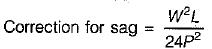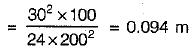Correct distance = 100 - 0.094 = 99.906 m

QUESTION: 6

Distance between two stations A and B can be precisely measured with one complete Gunter’s chain and one complete Engineer’s chain. The distance between A and B is

Solution:

Gunter’s chain is 66 ft long & Engineer’s chain is 100 ft long.
Distance between A & B
= 100 + 66 = 166 ft

QUESTION: 7

The downhill end of a 30 m tape is held 90 cm too low. What is the horizontal distance measured

Solution:

Correction for slope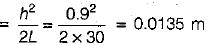∴ Horizontal distance
= 30 - 0.0135 = 29.9865 m

QUESTION: 8

The distance between the points measured along a slope is 428 m. If the angle of slope between the points is 8° than the horizontal distance between them is

Solution:

Horizontal distance = l cosθ
= 428 cos8° = 423.83 m

QUESTION: 9

A line of true length 500 m when measured by a 20 m tape is reported to be 502 m long. The correct length of the tape is

Solution:

Correct length of tape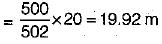QUESTION: 10

A 20 m chain was found to be 10 cm too long after chaining a distance of 2000 m. It was found to be 18 cm too long at the end of the day’s work after chaining a total distance of 4000 m. What is the true distance if the chain was correct before the commencement of the day’s work?

Solution:

For the first 2000 m, average error is,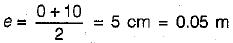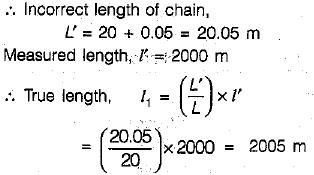For the next 2000 m, average error is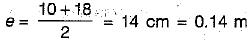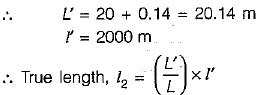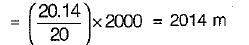Hence, true distance,